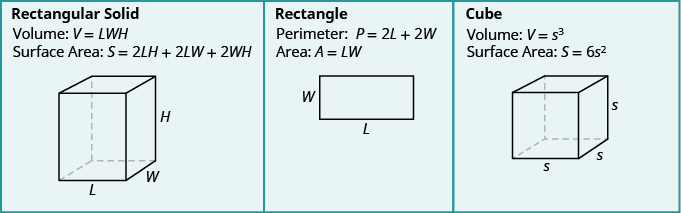## Key Concepts

• Volume and Surface Area of a Rectangular Solid
• $V=LWH$
• $S=2LH+2LW+2WH$
• Volume and Surface Area of a Cube
• $V={s}^{3}$
• $S=6{s}^{2}$
• Volume and Surface Area of a Sphere
• $V=\Large\frac{4}{3}\normalsize\pi {r}^{3}$
• $S=4\pi {r}^{2}$
• Volume and Surface Area of a Cylinder
• $V=\pi {r}^{2}h$
• $S=2\pi {r}^{2}+2\pi rh$
• Volume of a Cone
• For a cone with radius $r$ and height $h$ :Volume: $V=\Large\frac{1}{3}\normalsize\pi {r}^{2}h$

## Glossary

cone
A cone is a solid figure with one circular base and a vertex.
cube
A cube is a rectangular solid whose length, width, and height are equal.
cylinder
A cylinder is a solid figure with two parallel circles of the same size at the top and bottom.

## Summary of Geometry Formulas

The following charts summarize all of the formulas covered in this chapter.## Contribute!

Did you have an idea for improving this content? We’d love your input.